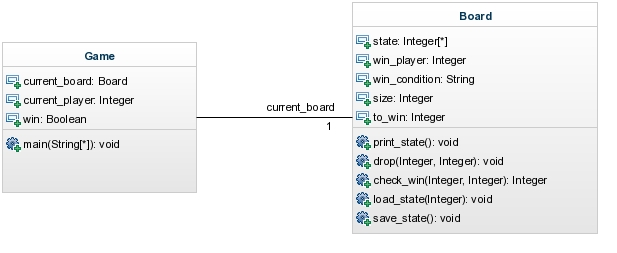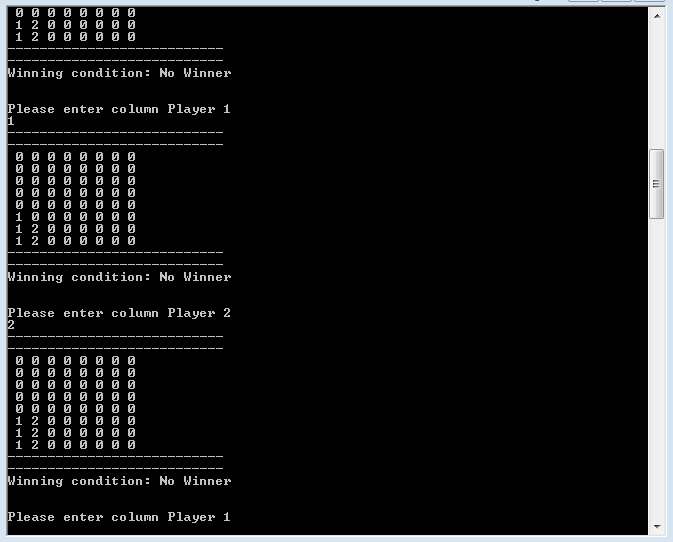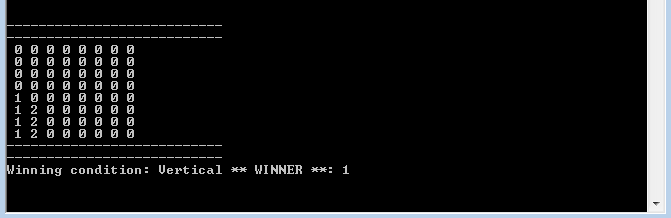# A Simple Text-based Connect4 Game

Oct 2014
Thu 09
0
0
0

## A couple of c# classes for the game of connect4

To the open source developer it's almost sacrilege but recently I've had the need to work with `c#`. To familiarise myself with the language and to grasp the differences with `c++`, I've written a very simple example program - the game of connect4 - which will be outlined in this post. To really mix things up, I moved away from Linux and OSX and worked with Visual Studio 2013 (express) on Windows 7 (albeit on a VM).

# Class Diagram

Firstly the (very) simple class diagram:Class diagram for a simple text-based connect4 game

The code will use a minimal class structure composing of two classes; `Game` and `Board`, with the simple association of a `Game` instance involving a single `Board` instance.

Before outlining each class, a few key concepts of the game:

• The state of the board is a 2D array of `int`
• Players are denoted by `int` values `1` or `2`
• A blank board space is denoted `0`
• Players take it in turns to `drop` their number into a column
• After each drop we check for a win condition. Doing this after each drop means we only have to check around the dropped column and row
• A win condition is achieved by connecting 4 (or the number denoted by `to_win`) horizontally, vertically or diagonally
• To mimic the board in real life, it is simply printed upside down (inverse row order)

# Game.cs

This class will serve as the entry point to the program containing the main method, let's take a look:

Game.cs

```using System;
using System.Collections.Generic;
using System.Linq;
using System.Text;

namespace Connect4
{
class Game
{
static void Main(string[] args)
{
Board current_board = new Board();
current_board.print_state();

bool win=false;
int current_player = 1;
while(!win)
{
int col;
while (!int.TryParse(scol.ToString(), out col))
{
Console.Write("Please enter column Player " + current_player + "\n");
}

int row = current_board.drop(col, current_player);
if (row == -1) continue;

win = current_board.check_win(col, row, current_player);

current_board.print_state();

current_player = (current_player == 1) ? 2 : 1;
}

current_board.print_state();
}
}
}
```

Stepping through this class we first encounter the instance of the `Board` class, which is initiated and its empty state printed:

```Board current_board = new Board();
current_board.print_state();
```

Next we enter the main game loop. We set the bool `win` to `false` and set the current player to `1`. Whilst we have not won (`!win`) we continue through a loop which consists of:

• asking a player for a column number
• check if column is valid
• dropping the player's number into that column
• checking for win condition

The checks for a valid column involve parsing the input `string` into an `int` using `int.TryParse`. This returns a bool denoting the success of the parse. Simply placing this into a loop forces the player to input a valid `int`:

```string scol = Console.ReadLine();
int col;
while (!int.TryParse(scol.ToString(), out col))
{
Console.Write("Please enter column Player " + current_player + "\n");
}
```

A second check involves ensuring a valid column is inputted. A column has to be above 0 and less than or equal to the size of the board. In addition, in a column is full the player is asked for another column. This functionality is implemented in the `Board` class by the `drop` method. This method returns an int which denotes the row of the drop. If the row is `-1` then the drop failed and we ask the player for another column by continuing the while loop.

```int row = current_board.drop(col, current_player);
if (row == -1) continue;
```

The code then moves to check for a win condition, returning a bool assigned to `win`:

```win = current_board.check_win(col, row, current_player);
```

If a win condition is not met we do not break out of the main loop and instead change player with a simple c-style inline if:

```current_player = (current_player == 1) ? 2 : 1;
```

# Board.cs

This class holds the functionality of the board independent of what game it is in.

Board.cs

```using System;
using System.Collections.Generic;
using System.Linq;
using System.Text;

namespace Connect4
{
class Board
{
int size = 8;
int to_win = 4;
int[,] state;
string win_condition = "No Winner";
int win_player = -1;
public Board()
{
state = new int[size, size];
reset();
}

public int drop(int col, int player)
{
// drop into col, return row or -1 if fail

col -= 1;
int row;
int success = -1;

if( col > size)
{
Console.Write("Board only has "+size+" columns \n");
return -1;
}

if (col < 0)
{
Console.Write("Really? Don't be so negative \n");
return -1;
}

for (row = 0; row < size; row++)
{
if(state[row,col] == 0)
{
state[row, col] = player;
success = row;
break;
}
}
if (row == size)
{
Console.Write("Column Full \n");
success = -1;
}
return success;

}

public bool check_win(int col, int row, int player)
{

col -= 1;
int[] check_flags = { -1, 1, -2, 2, -3, 3 };

//win count along each direction, forward and backward flags
int win_count, fflag, bflag;

//4 directions (h,v,d1,d2)
for (int direction = 0; direction < 4; direction++)
{
switch (direction)
{
case 0: //Horizontal
fflag = 0;
bflag = 0;
win_count = 1;
for (int i = 0; i < 6; i++)
{
int this_col = col + check_flags[i];

if (this_col < 0 || this_col >= size) continue;

if (state[row, this_col] == player)
{
win_count++;
}
else
{
if (check_flags[i] < 0) bflag = 1;
else fflag = 1;
}
if (bflag == 1 && fflag == 1) break;

}

if (win_count == to_win)
{
Console.Write("WINNER!! - Horizontal \n");
win_condition = "Horizontal";
win_player = player;
return true;
}
break;

case 1: //Vertical
fflag = 0;
bflag = 0;
win_count = 1;

for (int i = 0; i < 6; i++)
{
int this_row = row + check_flags[i];

if (this_row < 0 || this_row >= size) continue;

if (state[this_row, col] == player)
{
win_count++;
}
else
{
if (check_flags[i] < 0) bflag = 1;
else fflag = 1;
}
if (bflag == 1 && fflag == 1) break;

}

if (win_count == to_win)
{
Console.Write("WINNER!! - Vertical \n");
win_condition = "Vertical";
win_player = player;
return true;
}
break;

case 2: // == Diagonal

fflag = 0;
bflag = 0;
win_count = 1;

for (int i = 0; i < 6; i++)
{
int this_row = row + check_flags[i];
int this_col = col + check_flags[i];

if (this_row < 0 || this_row >= size) continue;
if (this_col < 0 || this_col >= size) continue;

if (state[this_row, this_col] == player)
{
win_count++;
}
else
{
if (check_flags[i] < 0) bflag = 1;
else fflag = 1;
}
if (bflag == 1 && fflag == 1) break;

}

if (win_count == to_win)
{
Console.Write("WINNER!! - Diagonal \n");
win_condition = "Diagonal (positive)";
win_player = player;
return true;
}
break;

case 3: // != Diagonal

fflag = 0;
bflag = 0;
win_count = 1;

for (int i = 0; i < 6; i++)
{
int this_row = row - check_flags[i];
int this_col = col + check_flags[i];

if (this_row < 0 || this_row >= size) continue;
if (this_col < 0 || this_col >= size) continue;

if (state[this_row, this_col] == player)
{
win_count++;
}
else
{
if (check_flags[i] < 0) bflag = 1;
else { fflag = 1; }
}
if (bflag == 1 && fflag == 1) break;

}

if (win_count == to_win)
{
Console.Write("WINNER!! - Diagonal \n");
win_condition = "Diagonal (negative)";
win_player = player;
return true;
}
break;

}

}

return false;

}

public void reset()
{
win_player = -1;
win_condition = "No Winner";
for (int row = 0; row < size; row++)
{
for (int col = 0; col < size; col++)
{
state[row,col] = 0;
}

}

}

public void print_state()
{
Console.Write("---------------------------\n");
Console.Write("---------------------------\n");
for (int row = size-1; row >= 0; row--)
{
string rstring = "";

for (int col = 0; col < size; col++)
{
rstring += " " + state[row,col];

}

Console.Write(rstring+"\n");

}
Console.Write("---------------------------\n");
Console.Write("---------------------------\n");

Console.Write("Winning condition: " + win_condition );
if (win_player != -1) { Console.Write(" ** WINNER **: " + win_player); }
Console.Write("\n\n\n");
}

~Board()
{

}

}

public void save_state()
{

}

}
}
```

Although this class is much longer than the `Game` class its functionality is relatively straightforward. Firstly the constructor initialises the state of the board:

```public Board()
{
state = new int[size, size];
reset();
}
```

where the reset function simply sets each entry in the state array to `0`.

The two methods of importance in the `Board` class are the `drop` and `check_win` functions. The `drop` method deals with checking for a valid column and finding the appropriate empty row to place the dropped player number:

```public int drop(int col, int player)
{
// drop into col, return row or -1 if fail

col -= 1;
int row;
int success = -1;

if( col > size)
{
Console.Write("Board only has "+size+" columns \n");
return -1;
}

if (col < 0)
{
Console.Write("Really? Don't be so negative \n");
return -1;
}

for (row = 0; row < size; row++)
{
if(state[row,col] == 0)
{
state[row, col] = player;
success = row;
break;
}
}
if (row == size)
{
Console.Write("Column Full \n");
success = -1;
}
return success;

}
```

The `check_win` method checks the board around the most recently dropped player number for a win condition. Checking around the last dropped row and column removes the need to check the whole board and significantly reduces running time:

```public bool check_win(int col, int row, int player)
{

col -= 1;
int[] check_flags = { -1, 1, -2, 2, -3, 3 };

//win count along each direction, forward and backward flags
int win_count, fflag, bflag;

//4 directions (h,v,d1,d2)
for (int direction = 0; direction < 4; direction++)
{
switch (direction)
{
case 0: //Horizontal
fflag = 0;
bflag = 0;
win_count = 1;
for (int i = 0; i < 6; i++)
{
int this_col = col + check_flags[i];

if (this_col < 0 || this_col >= size) continue;

if (state[row, this_col] == player)
{
win_count++;
}
else
{
if (check_flags[i] < 0) bflag = 1;
else fflag = 1;
}
if (bflag == 1 && fflag == 1) break;

}

if (win_count == to_win)
{
Console.Write("WINNER!! - Horizontal \n");
win_condition = "Horizontal";
win_player = player;
return true;
}
break;

case 1: //Vertical
fflag = 0;
bflag = 0;
win_count = 1;

for (int i = 0; i < 6; i++)
{
int this_row = row + check_flags[i];

if (this_row < 0 || this_row >= size) continue;

if (state[this_row, col] == player)
{
win_count++;
}
else
{
if (check_flags[i] < 0) bflag = 1;
else fflag = 1;
}
if (bflag == 1 && fflag == 1) break;

}

if (win_count == to_win)
{
Console.Write("WINNER!! - Vertical \n");
win_condition = "Vertical";
win_player = player;
return true;
}
break;

case 2: // == Diagonal

fflag = 0;
bflag = 0;
win_count = 1;

for (int i = 0; i < 6; i++)
{
int this_row = row + check_flags[i];
int this_col = col + check_flags[i];

if (this_row < 0 || this_row >= size) continue;
if (this_col < 0 || this_col >= size) continue;

if (state[this_row, this_col] == player)
{
win_count++;
}
else
{
if (check_flags[i] < 0) bflag = 1;
else fflag = 1;
}
if (bflag == 1 && fflag == 1) break;

}

if (win_count == to_win)
{
Console.Write("WINNER!! - Diagonal \n");
win_condition = "Diagonal (positive)";
win_player = player;
return true;
}
break;

case 3: // != Diagonal

fflag = 0;
bflag = 0;
win_count = 1;

for (int i = 0; i < 6; i++)
{
int this_row = row - check_flags[i];
int this_col = col + check_flags[i];

if (this_row < 0 || this_row >= size) continue;
if (this_col < 0 || this_col >= size) continue;

if (state[this_row, this_col] == player)
{
win_count++;
}
else
{
if (check_flags[i] < 0) bflag = 1;
else { fflag = 1; }
}
if (bflag == 1 && fflag == 1) break;

}

if (win_count == to_win)
{
Console.Write("WINNER!! - Diagonal \n");
win_condition = "Diagonal (negative)";
win_player = player;
return true;
}
break;

}

}

return false;

}
```

The function above loops through the 4 possible directions (horizontal, vertical, positive diagonal and negative diagonal) checking forwards and backwards around the last dropped row and column. If an empty state is found or a entry containing the alternative player number then we break out of that particular check. To implement this, the array `check_flags` is used:

```int[] check_flags = { -1, 1, -2, 2, -3, 3 };
```

Starting and the current droped position, we can use the above to continual work away from our position checking as we go. As we are checking both forwards and backwards we must keep track of both directions using the `bflag` and `fflag`. When both these flags are set to `1` for a particular direction we can break out and begin to check the next direction. If at anytime the `win_count` equals `to_win` we return `true` and note the player number and winning direction.

# Use

Below are screen shots of the code in action. To expand this example to include more players or even more dimensions (i.e. a 3D board) should be possible.Sample output from the connect4 gameWinning condition met

# Coming soon

With this engine in place, I plan to code up a simple 2D visualisation of the game with mouse interaction. Watch this space...